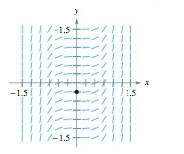Chapter 8.3, Problem 38E

Chapter
Section
Textbook Problem

# Slope Field In Exercises 39 and 40, a differential equation, a point, and a slope field are given, (a) Sketch two approximate solutions of the differential equation on the slope field, one of which passes through the given point. (To print an enlarged copy of the graph, go to MathGraphs.com.) (b) Use integration and the given point to find the particular solution of the differential equation and use a graphing utility to graph the solution. Compare the result with the sketch in part (a) that passes through the given point. d y d x = sec 2 x tan 2 x , ( 0 , 1 4 )(a)

To determine

To calculate: The graph of the two approximate solutions of the provided differential equation on the slope field such that one of them should pass through the given point.

Explanation

Given:

The provided differential equation is as follows;

dydx=sec2xtan2x,(0,14)

And the slope field,

Calculation:

First consider the provided differential equation,

dydx=sec2xtan2x,(0,14)

Now solve this differential equation as shown below,

dydx=sec2xtan2xdy=sec2xtan2xdx</

(b)

To determine

To calculate: The particular solution and graph of the particular solution for the provided differential equation using graphing utility.

### Still sussing out bartleby?

Check out a sample textbook solution.

See a sample solution

#### The Solution to Your Study Problems

Bartleby provides explanations to thousands of textbook problems written by our experts, many with advanced degrees!

Get Started

#### Find more solutions based on key concepts